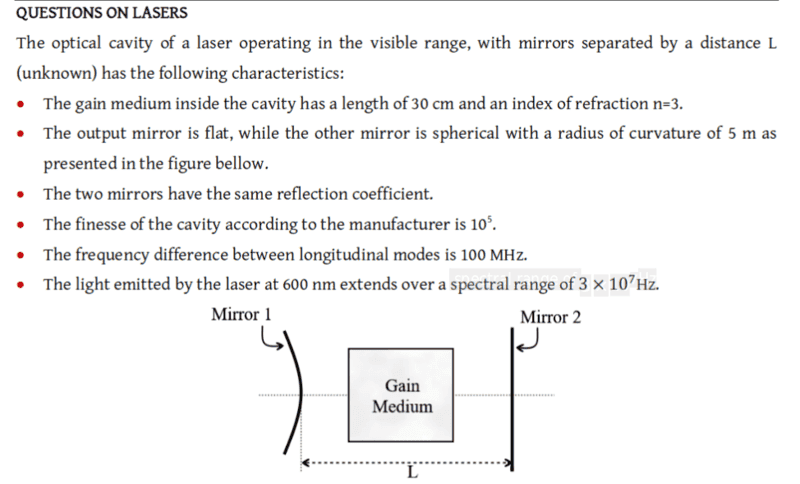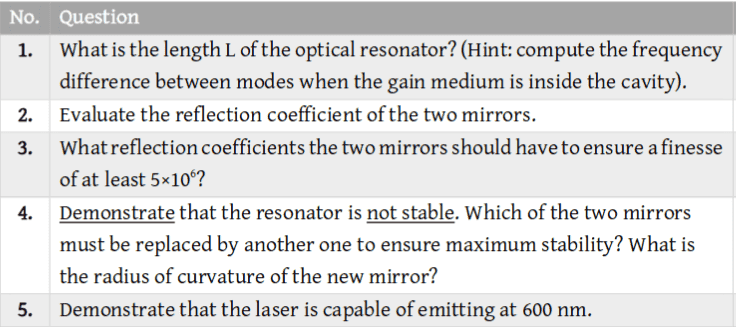# Wavelength of a laser within an optical cavity

1. The problem statement, all variables and given/known date## Homework Equations

$$\delta v=\frac{c}{2nL} \:$$
$$N=\frac{\Delta v}{\delta v}=\frac{2nL\Delta v}{c} \:$$

## The Attempt at a Solution

I am having trouble with question 5, but have come to realise I think my cavity length is wrong but I cant see how.

Here my working for question 1

Assuming that the medium is in the middle at equal distance from each mirror, then I could assume that ##L=L_{m}+2x## so subsituiting this into equation 1.

$$\delta v=\frac{c}{2nL}=\frac{c}{2n(L_{m}+2x)}$$

by rearranging the equation for x

$$x=\left(\frac{c}{\delta \:v}\cdot \:\frac{1}{2n}-Lm\right)\cdot \frac{1}{2}$$

so subbing ##x=0.1## I make the cavity length ##L=L_{m}+2(0.1)=0.5m##.

Which seem to reasonable to me. But if I plug this length of the cavity into  and using the spectral range of ##3\times 10^{7}## I make the number of modes 0.3 which cant be correct.

So the reason I want to workout the number of modes is so I can verify the ##600nm## via the equation ##\lambda=2\times L_{c}/N##, which dose not give the 600nm so either my cavity is length is wrong or my understanding is wrong.

Any advice would be much appreciated.